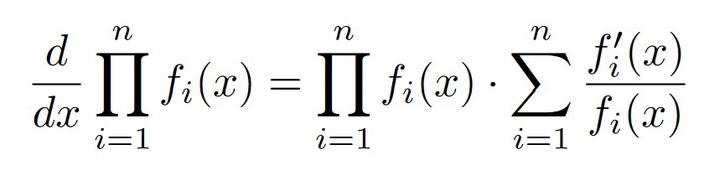# Elegant Derivatives of Large ProductsAn ongoing research project on modeling computer security has thrown me back into calculus for the first time since my undergraduate differential equations course in 2014, which as an aside was the only college course that forced me to buy the professor’s custom textbook just to get access to homework problems. (I’m still mad about that.) Anyway, my research led me to take the derivative of an expression of the form:

$$\prod_{i=1}^n f_i(x)$$

For the uninitiated, $\prod$ is a mathematical symbol meaning “product” (and is analogous to the $\sum$ symbol meaning “sum”). It says to look at each $f_i$ term as $i$ goes from $1, 2, \ldots, n$ and multiply them all together:

$$\prod_{i=1}^n f_i(x) = f_1(x) \times f_2(x) \times \cdots \times f_n(x)$$

Taking this derivative is something high-school me was much better prepared for than current postdoc me, but after some head scratching I found the elegant solution:

$$\frac{d}{dx} \prod_{i=1}^n f_i(x) = \prod_{i=1}^n f_i(x) \cdot \sum_{i=1}^n \frac{f_i'(x)}{f_i(x)}$$

As is common in mathematics, there’s a direct but tedious way to get this answer and another more elegant way to get the same thing. I’m not the first to derive this solution, but I’m writing it up for posterity because I will inevitably forget this trick in a few days. A PDF of the two derivations without all the handholding I’m about to do is available here.

## Method 1: Product Rule

I had to sweep away years of mental cobwebs to remember the product rule for derivatives. My expression is a product, after all, so why not start there? The product rule states:

$$\frac{d}{dx}(u \cdot v) = u' \cdot v + u \cdot v'$$

This works for the product of two terms, but $\prod_{i=1}^n f_i(x)$ has $n$ terms. Let’s try peeling off terms one-by-one, starting with $f_1(x)$, and then applying the product rule.

\begin{align*} \frac{d}{dx} \prod_{i=1}^n f_i(x) &= \frac{d}{dx} \left(f_1(x) \cdot \prod_{i=2}^n f_i(x)\right) \\ &= f_1'(x) \cdot \prod_{i=2}^n f_i(x) + f_1(x) \cdot \frac{d}{dx} \left(\prod_{i=2}^n f_i(x)\right) \end{align*}

That’s progress, but now we have to repeat the process to deal with the $\frac{d}{dx} \left(\prod_{i=2}^n f_i(x)\right)$ term, peeling off $f_2(x)$ and applying the product rule again:

\begin{align*} \frac{d}{dx} \prod_{i=1}^n f_i(x) &= f_1'(x) \cdot \prod_{i=2}^n f_i(x) + f_1(x) \cdot \frac{d}{dx} \left(\prod_{i=2}^n f_i(x)\right) \\ &= f_1'(x) \cdot \prod_{i=2}^n f_i(x) + f_1(x) \\ &\quad \cdot \left(f_2'(x) \cdot \prod_{i=3}^n f_i(x) + f_2(x) \cdot \frac{d}{dx} \left(\prod_{i=3}^n f_i(x)\right)\right) \end{align*}

This expression’s going to have more layers than an ogre! If we were to do the peel-and-product rule strategy $n-1$ times, we would arrive at:

\begin{align*} \frac{d}{dx} \prod_{i=1}^n f_i(x) &= f_1'(x) \cdot \prod_{i=2}^n f_i(x) + f_1(x) \\ &\quad \cdot \left(f_2'(x) \cdot \prod_{i=3}^n f_i(x) + f_2(x) \right. \\ &\quad \cdot \left(f_3'(x) \cdot \prod_{i=4}^n f_i(x) + f_3(x) \cdots + f_{n-2}(x) \right. \\ &\quad \left. \left. \cdot \left(f_{n-1}'(x) \cdot f_n(x) + f_n'(x) \cdot f_{n-1}(x)\right) \cdots \right) \right) \end{align*}

Do you see the pattern? If we distribute the $f_i(x)$ terms at the end of each of the lines above into the parentheses that immediately follow,

\begin{align*} \frac{d}{dx} \prod_{i=1}^n f_i(x) &= f_1'(x) \cdot \prod_{i=2}^n f_i(x) + f_2'(x) \cdot f_1(x) \cdot \prod_{i=3}^n f_i(x) \\ &\quad + f_3'(x) \cdot f_1(x) \cdot f_2(x) \cdot \prod_{i=4}^n f_i(x) \\ &\quad + f_{n-1}'(x) \cdot f_1(x) \cdots f_{n-2}(x) \cdot f_n(x) \\ &\quad + f_n'(x) \cdot f_1(x) \cdots f_{n-1}(x) \end{align*}

We’re adding up a bunch of terms, each of which is a derivative $f_i'(x)$ multiplied by all the other $f_j(x)$’s, where $j \neq i$. Landing this messy, notation-heavy plane, we can rewrite this long sum as

$$\frac{d}{dx} \prod_{i=1}^n f_i(x) = \sum_{i=1}^n f_i'(x) \cdot \frac{\prod_{j=1}^n f_j(x)}{f_i(x)} = \prod_{i=1}^n f_i(x) \cdot \sum_{i=1}^n \frac{f_i'(x)}{f_i(x)},$$

which is the solution we came for.

## Method 2: Leveraging Logarithms

Mathematicians crave elegance, and Method 1 wasn’t that. We had to keep keen eyes out for patterns as they emerged and carefully distribute/rearrange many terms to get the solution. This second method will be much cleaner, but as many clever methods do, it begins with an unintuitive step.

Let $F(x) = \prod_{i=1}^n f_i(x)$. Taking the natural logarithm of both sides,

$$\ln(F(x)) = \ln\left(\prod_{i=1}^n f_i(x)\right) = \sum_{i=1}^n \ln(f_i(x)),$$

where the last equality follows from the fact that the logarithm of a product is equal to the sum of logarithms. The derivative of the natural logarithm is:

$$\frac{d}{dx}\ln(x) = \frac{1}{x}$$

Using chain rule to take the derivative of both sides of the above equality,

\begin{align*} \frac{d}{dx}\ln(F(x)) &= \frac{d}{dx}\sum_{i=1}^n \ln(f_i(x)) \\ \frac{1}{F(x)} \cdot \frac{dF}{dx} &= \sum_{i=1}^n \frac{1}{f_i(x)} \cdot f_i'(x) \\ \frac{dF}{dx} &= F(x) \cdot \sum_{i=1}^n \frac{f_i'(x)}{f_i(x)} \end{align*}

If we plug $F(x) = \prod_{i=1}^n f_i(x)$ back in, out pops the solution:

$$\frac{d}{dx} \prod_{i=1}^n f_i(x) = \prod_{i=1}^n f_i(x) \cdot \sum_{i=1}^n \frac{f_i'(x)}{f_i(x)}$$

## Concluding Thoughts

Having initially stumbled upon Method 1, I was surprised that this derivative came out so beautifully in the end. Credit where it’s due: I adapted the elegant Method 2 from this post regarding infinite products. I have some technical notes at the end of the linked PDF about when Method 2 breaks down, though I believe Method 1 does not have any of these problems.

Hopefully this was fun (and if it wasn’t, I doubt you read this far 😉). This is one of many examples in mathematics of finding multiple solutions to the same problem and learning something different each time. Next time you emerge victorious from a tedious derivation, see if you can find a clever alternative!##### Joshua J. Daymude
###### Assistant Professor, SCAI & CBSS

I am a Christian and assistant professor in computer science studying collective emergent behavior and programmable matter through the lens of distributed computing, stochastic processes, and bio-inspired algorithms. I also love gaming and playing music.